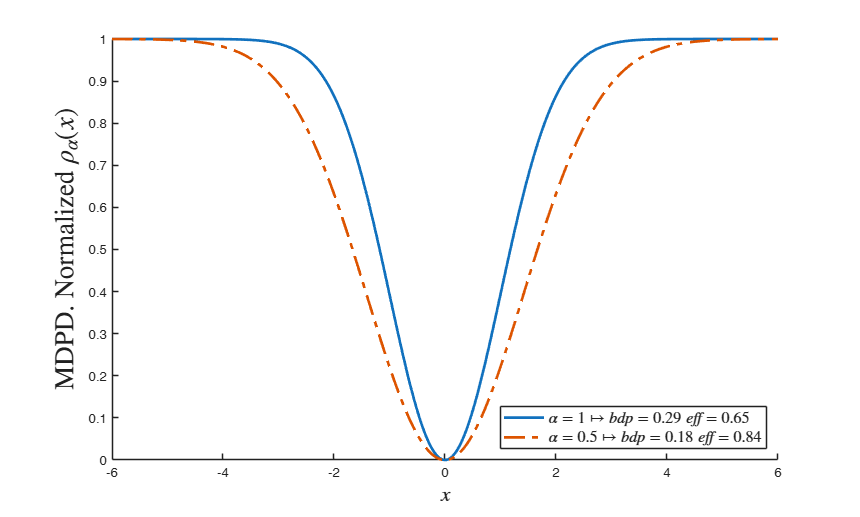# PDrho

PDrho computes rho function for minimum density power divergence estimator

## Syntax

• rhoPD=PDrho(u,alpha)example

## Description

 rhoPD =PDrho(u, alpha) Plot of rho function.

## Examples

expand all

### Plot of rho function.

close all
x=-6:0.01:6;
alpha=1;
rhoPD=PDrho(x,alpha);
plot(x,rhoPD,'LineWidth',2)
xlabel('$u$','Interpreter','Latex')
ylabel('$\rho (u,1)$','Interpreter','Latex')

## Related Examples

expand all

###Compare two rho functions for 2 different values of alpha.

In the first we fix the bdp (value of efficiency is automatically given), while in the second we find the efficiency (the value of bdp is automatically given)

close all
x=-6:0.01:6;
lwd=2;
alpha1=1;
bdp1=1-1/sqrt(1+alpha1);
eff1=(sqrt(1+2*alpha1)/(1+alpha1))^3;
hold('on')
rhoPD=PDrho(x,alpha1);
plot(x,rhoPD,'LineStyle','-','LineWidth',lwd)
alpha2=0.5;
bdp2=1-1/sqrt(1+alpha2);
eff2=(sqrt(1+2*alpha2)/(1+alpha2))^3;
rhoPD=PDrho(x,alpha2);
plot(x,rhoPD,'LineStyle','-.','LineWidth',lwd)
xlabel('$x$','Interpreter','Latex','FontSize',16)
ylabel('MDPD. Normalized $\rho_\alpha(x)$','Interpreter','Latex','FontSize',20)
legend({['$\alpha=' num2str(alpha1) '\mapsto bdp=' num2str(bdp1,2) '\; eff=' num2str(eff1,2) '$'], ...
['$\alpha=' num2str(alpha2) '\mapsto bdp=' num2str(bdp2,2) '\; eff=' num2str(eff2,2) '$']},...
'Interpreter','Latex','Location','SouthEast','FontSize',12)## Input Arguments

### u — scaled residuals or Mahalanobis distances. Vector.

n x 1 vector containing residuals or Mahalanobis distances for the n units of the sample

Data Types: single| double

### alpha — tuning parameter. Scalar.

Scalar in the interval (0,1] which controls the robustness/efficiency of the estimator (beta in regression or mu in the location case ...). The greater is alpha the greater is the bdp and smaller is the efficiency.

Data Types: single| double

## Output Arguments

### rhoPD —Minimum density power divergence rho function associated to the residuals or Mahalanobis distances for the n units of the sample. n -by- 1 vector

function PDrho transforms vector u as follows $PDrho(u,alpha)= 1-\exp(-\alpha (u^2/2));$